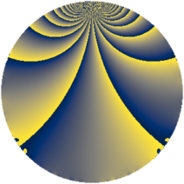# Properties

 Label 1323.2.pLevel $1323$ Weight $2$ Character orbit 1323.p Rep. character $\chi_{1323}(80,\cdot)$ Character field $\Q(\zeta_{6})$ Dimension $106$ Sturm bound $336$

# Related objects

## Defining parameters

 Level: $$N$$ $$=$$ $$1323 = 3^{3} \cdot 7^{2}$$ Weight: $$k$$ $$=$$ $$2$$ Character orbit: $$[\chi]$$ $$=$$ 1323.p (of order $$6$$ and degree $$2$$) Character conductor: $$\operatorname{cond}(\chi)$$ $$=$$ $$21$$ Character field: $$\Q(\zeta_{6})$$ Sturm bound: $$336$$

## Dimensions

The following table gives the dimensions of various subspaces of $$M_{2}(1323, [\chi])$$.

Total New Old
Modular forms 384 106 278
Cusp forms 288 106 182
Eisenstein series 96 0 96

## Trace form

 $$106q + 52q^{4} + O(q^{10})$$ $$106q + 52q^{4} - 6q^{10} - 82q^{16} + 3q^{19} + 72q^{22} - 49q^{25} + 39q^{31} - 14q^{37} + 12q^{40} + 46q^{43} + 42q^{46} - 36q^{52} + 20q^{58} - 15q^{61} - 280q^{64} - 72q^{67} - 51q^{73} + 92q^{79} - 18q^{82} + 4q^{85} + 38q^{88} + 138q^{94} + O(q^{100})$$

## Decomposition of $$S_{2}^{\mathrm{new}}(1323, [\chi])$$ into newform subspaces

The newforms in this space have not yet been added to the LMFDB.

## Decomposition of $$S_{2}^{\mathrm{old}}(1323, [\chi])$$ into lower level spaces

$$S_{2}^{\mathrm{old}}(1323, [\chi]) \cong$$ $$S_{2}^{\mathrm{new}}(21, [\chi])$$$$^{\oplus 6}$$$$\oplus$$$$S_{2}^{\mathrm{new}}(63, [\chi])$$$$^{\oplus 4}$$$$\oplus$$$$S_{2}^{\mathrm{new}}(147, [\chi])$$$$^{\oplus 3}$$$$\oplus$$$$S_{2}^{\mathrm{new}}(189, [\chi])$$$$^{\oplus 2}$$$$\oplus$$$$S_{2}^{\mathrm{new}}(441, [\chi])$$$$^{\oplus 2}$$